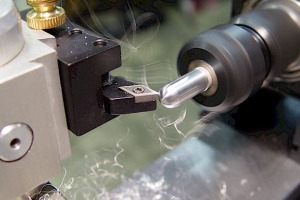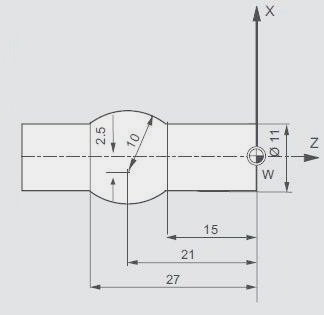# CNC Program Example G03 Circular Interpolation

Now a day CAD and CAM software make our lives just easier and easier, you just not have to calculate a single point in drawing, every thing is just ready. But it is a good practice to know actually how the calculations are being done, if not all but some which are necessary for a CNC programmer.CNC Program Example G03 Circular Interpolation

The calculation of G02 G03 circular interpolation values is a good technique to learn. This CNC program tells not all but a little of course enough for the beginner CNC programmer and CNC students.

## CNC Program With G03 Circular InterpolationCNC Program Example G03 Circular Interpolation

```N1 G00 X0 Z0
N2 G01 X11
N3 G01 Z-15
N4 G03 X11 Z-27 I-8 K-6```

### Description

The I and K for the G03.

### I Calculation for G03 Circular Interpolation

I is the distance from the arc start point to arc center point in x-axis. This value is not given in diameter but as a radius value, so

• Find the start diameter it is 11
• Now make it radius value by dividing it by 2 such as 11 / 2 = 5.5
• This is a easy example in way that the arc center point distance from the workpiece center point is given other wise we have to calculate it.
• So now just add 2.5 in 5.5 earlier calculated 2.5 + 5.5 = 8
• This is the value of I 8 this is the distance from the arc start point to the ace center point.

### K Calculation for G03 Circular Interpolation

K is the distance from the arc start point to arc center point in z-axis.

• In this example it is just easy as you just have to subtract 21 from 15 such as 21 – 15 = 6.
• So the value from the arc start point to the arc center point in z-axis is 6